# Magnetic Reconnection

Suppose that the resonant layer lies in a constant-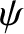response regime. According to Equation (5.33), the perturbed helical magnetic flux in the layer takes the form: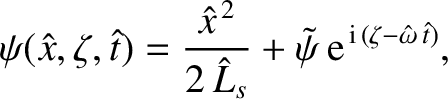(5.125)

where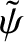is a complex constant. Of course, the physical flux is the real part of the previous expression; that is,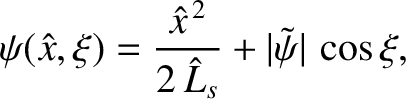(5.126)

where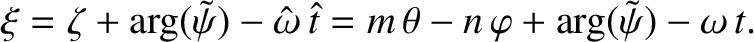(5.127)

Now, it is clear from Equation (5.1) that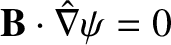. In other words, the contours of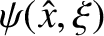map out the perturbed magnetic flux-surfaces in the vicinity of the resonant layer. Let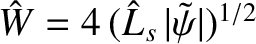. Equation (5.126) can be written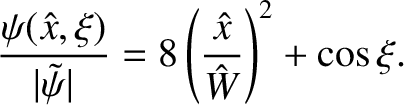(5.128)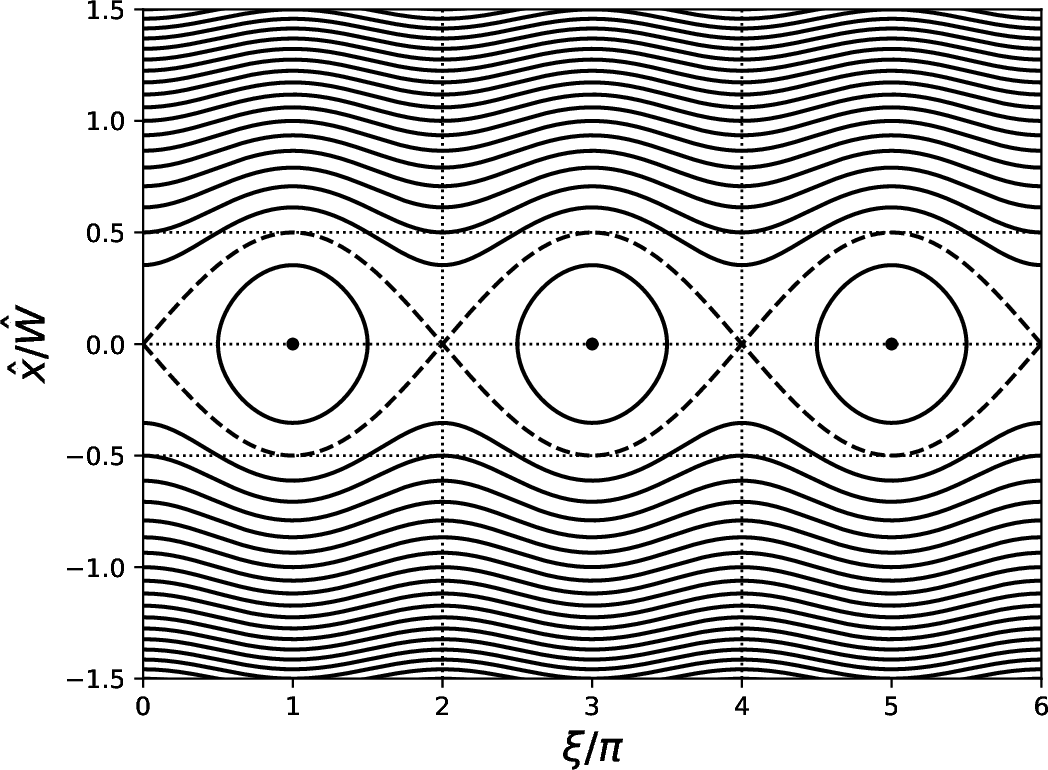Figure 5.7 shows the contours ofspecified by the previous equation. It can be seen that the tearing mode has changed the topology of the magnetic field in the immediate vicinity of the rational surface,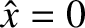. In fact, as the tearing mode grows in amplitude (i.e., as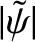increases), magnetic field-lines pass through the magnetic “X-points” (which are located at,, where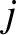is an integer), at which time they break (or “tear”) and then reconnect to form new field-lines that do not extend over all values of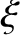. The magnetic field-line that forms the boundary between the unreconnected and reconnected regions is known as the magnetic separatrix, and corresponds to the contour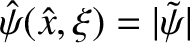. The reconnected regions within the magnetic separatrix are termed magnetic islands. The tearing mode clearly generates a chain of magnetic islands, centered on the rational surface, with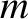periods in the poloidal direction, and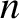periods in the toroidal direction, which propagates in the laboratory frame at the helical phase velocity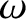. The maximum full (as opposed to half) radial width of the magnetic separatrix is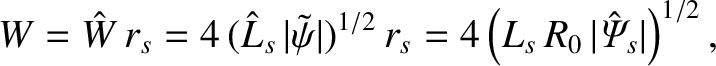(5.129)

where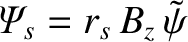is the reconnected magnetic flux defined in Equation (3.72), and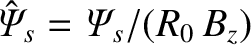.

Finally, our reduced drift-MHD model, (5.10)–(5.13), contains many term of the general form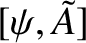, where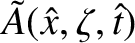is a perturbed quantity. According to Equation (5.126), such terms can be written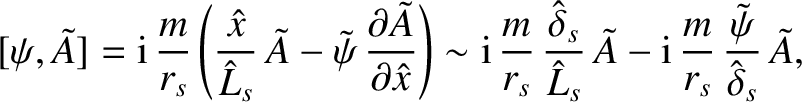(5.130)

where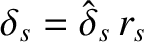is the radial width of the linear layer. Now, linear layer theory is only valid when the second term on the extreme right side of the previous equation is negligible compared to the first (because the second term is quadratic in perturbed quantities, whereas the first is linear). In other words, we require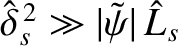, which reduces to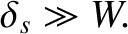(5.131)

[See Equation (5.129).] We conclude that the linear resonant response theory presented in this chapter is only valid when the radial width of the magnetic island chain that develops in the vicinity of the rational surface is much less than the radial width of the linear layer. Given that linear layers are very narrow in conventional tokamak plasmas (see Chapter 6), this is a stringent constraint.